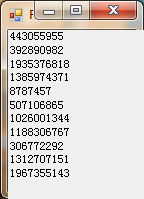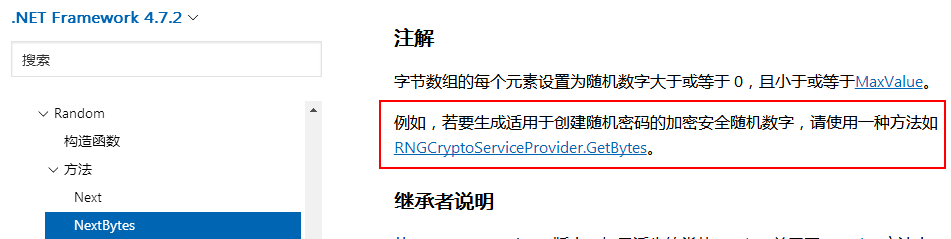# VB.NET学习笔记：使用Random类生成随机数（不重复、数字、字母）

VB6.0升级到VB.NET后，发现随机数函数也发生了变化，在VB.NET中Random类是一种能够产生满足某些随机性统计需求的数字序列的伪随机数生成器。

1、构造函数：
（1）、Random()

（2）、Random(Int32)

2、方法：
（1）、Next：返回一个随机整数。
A、无参数：Next()——返回一个非负随机整数。
B、一个参数：Next(maxValue) ——返回一个小于所指定最大值的非负随机整数。

C、两个参数：Next(minValue,maxValue)——返回在指定范围内的任意整数。

（2）、NextBytes：用随机数填充指定字节数组的元素。
NextBytes(buffer)

（3）、NextDouble：返回一个大于或等于 0.0 且小于 1.0 的随机双精度型浮点数。

3、使用方法：

Random类生成随机数是伪随机数，所以在Random的内部产生机制中还是有一定规律的，并非是真正意义上的完全随机。 如果种子值相同则每次均产生相同的随机数。如下面代码在构造函数中指定了种子值：

Private Sub Form1_Load(sender As Object, e As EventArgs) Handles MyBase.Load
Dim rand As Random = New Random(66)
For i As Integer = 0 To 10
Me.Label1.Text = Me.Label1.Text & rand.Next() & Environment.NewLine
Next
End SubPublic Class Form1
''' <summary>
''' 通过RNGCryptoServiceProvider获取种子值
''' </summary>
''' <returns></returns>
Private Shared Function GetRandomSeed() As Integer
Dim bytes As Byte() = New Byte(3) {}
Dim rng As System.Security.Cryptography.RNGCryptoServiceProvider = New System.Security.Cryptography.RNGCryptoServiceProvider()
rng.GetBytes(bytes)
Return BitConverter.ToInt32(bytes, 0)
End Function

''' <summary>
''' 通过Guid获取种子值
''' </summary>
''' <returns></returns>
Private Shared Function GetRandomSeedbyGuid() As Integer
'Return New Guid().GetHashCode()
Return Guid.NewGuid().GetHashCode()
End Function

''' <summary>
''' 默认种子值生成随机数
''' </summary>
''' <param name="sender"></param>
''' <param name="e"></param>
Private Sub BtnTime_Click(sender As Object, e As EventArgs) Handles BtnTime.Click
Dim rand As Random = New Random()
Me.Label1.Text = ""
For i As Integer = 0 To 10
Me.Label1.Text = Me.Label1.Text & rand.Next() & Environment.NewLine
Next
End Sub

''' <summary>
''' RNGCryptoServiceProvider种子生成随机数
''' </summary>
''' <param name="sender"></param>
''' <param name="e"></param>
Private Sub BtnRNG_Click(sender As Object, e As EventArgs) Handles BtnRNG.Click
Dim rand As Random = New Random(GetRandomSeed())
Me.Label1.Text = ""
For i As Integer = 0 To 10
Me.Label1.Text = Me.Label1.Text & rand.Next() & Environment.NewLine
Next
End Sub

''' <summary>
''' Guid种子生成随机数
''' </summary>
''' <param name="sender"></param>
''' <param name="e"></param>
Private Sub BtnGuid_Click(sender As Object, e As EventArgs) Handles BtnGuid.Click
Dim rand As Random = New Random(GetRandomSeedbyGuid())
Me.Label1.Text = ""
For i As Integer = 0 To 10
Me.Label1.Text = Me.Label1.Text & rand.Next() & Environment.NewLine
Next
End Sub
End Class


Public Function GetAlphanumeric() As String
Dim str As String = "0123456789abcdefghijklmnopqrstuvwxyzABCDEFGHIJKLMNOPQRSTUVWXYZ"
Dim rnd As New Random()
'返回数字
'Return rnd.[Next](10).ToString()
'返回小写字母
Return str.Substring(10 + rnd.[Next](26), 1)
'返回大写字母
'Return str.Substring(36 + rnd.[Next](26), 1)
'返回大小写字母混合
'Return str.Substring(10 + rnd.[Next](52), 1)
'返回小写字母和数字混合
'Return str.Substring(0 + rnd.[Next](36), 1)
'返回大写字母和数字混合
'Return str.Substring(0 + rnd.[Next](36), 1).ToUpper()
'返回大小写字母和数字混合
'Return str.Substring(0 + rnd.[Next](61), 1)
End Function


1、 从N个数中随机取出一个数，与数组第N个数调换；
2、 从前N-1个数中随机取出一个数，与第（N-1）个数调换；
……

Public Function GetRandomSequence(ByVal total As Integer) As Integer()
Dim sequence As Integer() = New Integer(total - 1) {}
Dim output As Integer() = New Integer(total - 1) {}

For i As Integer = 0 To total - 1
sequence(i) = i
Next

Dim random As Random = New Random()
Dim _End As Integer = total - 1

For i As Integer = 0 To total - 1
Dim num As Integer = random.[Next](0, _End + 1)
output(i) = sequence(num)
sequence(num) = sequence(_End)
_End -= 1
Next

Return output
End Function


06-1312-2366
08-09509
09-173646
06-29
03-18
12-31
05-25214